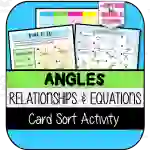Math > Geometry | Grade 7 | Activities

# Angle Relationships & Equations CARD SORTAttributes
Subject

Geometry

Types

Activities

File

PDF

Editable
No
RatingThis resource was developed to meet the requirements of the 7th Grade Geometry Standard below:

CCSS.MATH.CONTENT.7.G.B.5
Use facts about supplementary, complementary, vertical, and adjacent angles in a multi-step problem to write and solve simple equations for an unknown angle in a figure.

## What's Included

This resource contains:

1) Angle Relationships & Equations CARD SORT Activity (printable & version for Google Slides)

--A brief introductory activity that will help students understand the task and promote class discussion.

--Students will cut, sort, and paste 20 different angle relationship images into the following categories:

x°+y°=90° (for complementary angles)

x°+y°=180° (for supplementary angles)

x°+y°=z° (for adjacent angles that have neither a complementary nor supplementary relationship)

x°=y° (for vertical angles)

Note: This activity does NOT require students to solve equations; it is meant to be an activity to help them identify the TYPE of equation that first needs to be set up before attempting to solve the equation.

## Resource Tags

angle relationships equations card sort google slides

## 0 Reviews

### you may also like...

Check out these other great products